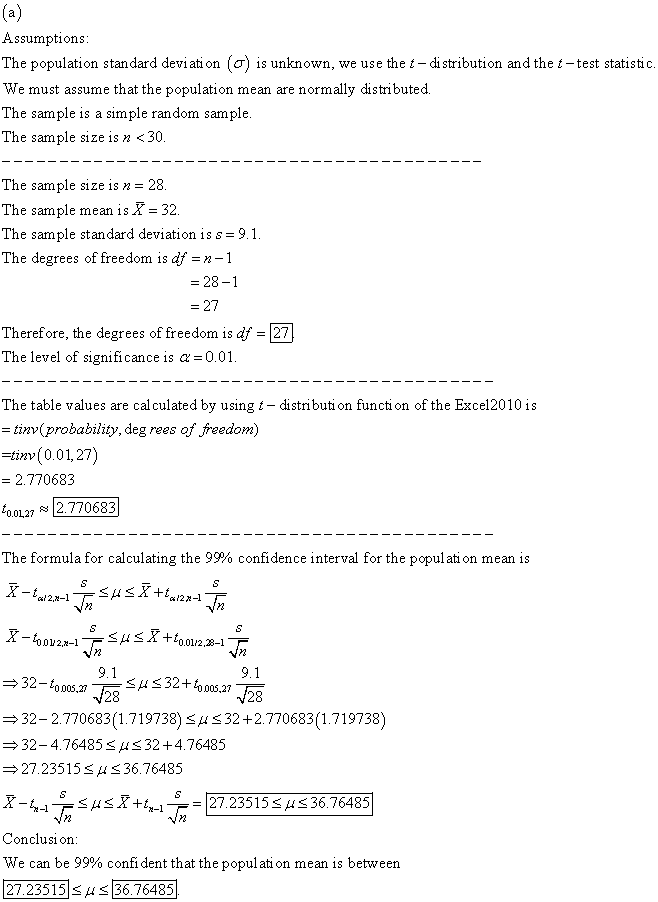##### Question

In: Statistics and Probability

# Construct a 99​% confidence interval to estimate the population mean using the data below. What assumptions...

Construct a 99​% confidence interval to estimate the population mean using the data below. What assumptions need to be made about this​ population?

 x overbar =32 s=9.1 n=28

## Solutions

##### Expert Solution## Related Solutions

##### Construct a 99​% confidence interval to estimate the population mean using the data below. X (overbar)...
Construct a 99​% confidence interval to estimate the population mean using the data below. X (overbar) = 22 s = 3.2 n = 13 What assumptions need to be made about this​ population? The 99​% confidence interval for the population mean is from a lower limit of __ to an upper limit of __. (round to two decimal places as needed.)
##### Construct a 95?% confidence interval to estimate the population mean using the following data. What assumptions...
Construct a 95?% confidence interval to estimate the population mean using the following data. What assumptions need to be made to construct this? interval? x?=93 ?=15 n=17 With 95?% ?confidence, when n=17 the population mean is between the lower limit of __ and the upper limit of __
##### Construct a 90​% confidence interval to estimate the population mean using the accompanying data. What assumptions...
Construct a 90​% confidence interval to estimate the population mean using the accompanying data. What assumptions need to be made to construct this​ interval? x=59 σ=15 n=17 What assumptions need to be made to construct this​ interval? A. The population is skewed to one side. B. The sample size is less than 30. C. The population must be normally distributed. D. The population mean will be in the confidence interval. With 90​% ​confidence, when n=17​, the population mean is between...
##### Construct a 95% confidence interval to estimate the population mean using the following data. What assumptions...
Construct a 95% confidence interval to estimate the population mean using the following data. What assumptions need to be made to construct this​ interval? x(over bar x)= 83   s=19    n=16 What assumptions need to be made to construct this​ interval? A. The population is skewed to one side. B. The sample size is less than 30. C. The population mean will be in the confidence interval. D. The population must be normally distributed. With 95​% ​confidence, when n=16 the population...
##### Construct a 95% confidence interval to estimate the population mean using the data below. What assumptions need to be made about this population?
Construct a 95% confidence interval to estimate the population mean using the data below. What assumptions need to be made about this population? x̄ = 33 s= 9.9 n=27 The 95% confidence interval for the population mean is from a lower limit of _______  to an upper limit of _______ .
##### construct a 99% confidence interval to estimate the population mean using the data below x=36 sigma=9...
construct a 99% confidence interval to estimate the population mean using the data below x=36 sigma=9 n=38
##### Construct a 95​% confidence interval to estimate the population mean using the data below. x̅= 46...
Construct a 95​% confidence interval to estimate the population mean using the data below. x̅= 46 σ=12 n= 41 With 95​% confidence, when n=41 the population mean is between a lower limit of ___ and upper limit of ___
##### Microsoft MiniTab Assignment Construct and interpret the 99 % confidence interval estimate of the population mean...
Microsoft MiniTab Assignment Construct and interpret the 99 % confidence interval estimate of the population mean “WaitTime” for all customers. Type in your explanation below the output from Minitab. Construct and interpret the 99% confidence interval estimate of the population proportion of male customers. Type in your explanation below the output from Minitab. Is the mean “Income” for all customers no more than \$38,000? Perform the appropriate hypothesis test using alpha = 0.05. Type in your explanation below the output...
##### Construct a? 95% confidence interval to estimate the population proportion using the data below. x =...
Construct a? 95% confidence interval to estimate the population proportion using the data below. x = 29 n = 90 N = 500 The? 95% confidence interval for the population proportion is? (_,_).
##### Construct a 90% confidence interval to estimate the population mean using the data below. x=19 s=4.2...
Construct a 90% confidence interval to estimate the population mean using the data below. x=19 s=4.2 n=14